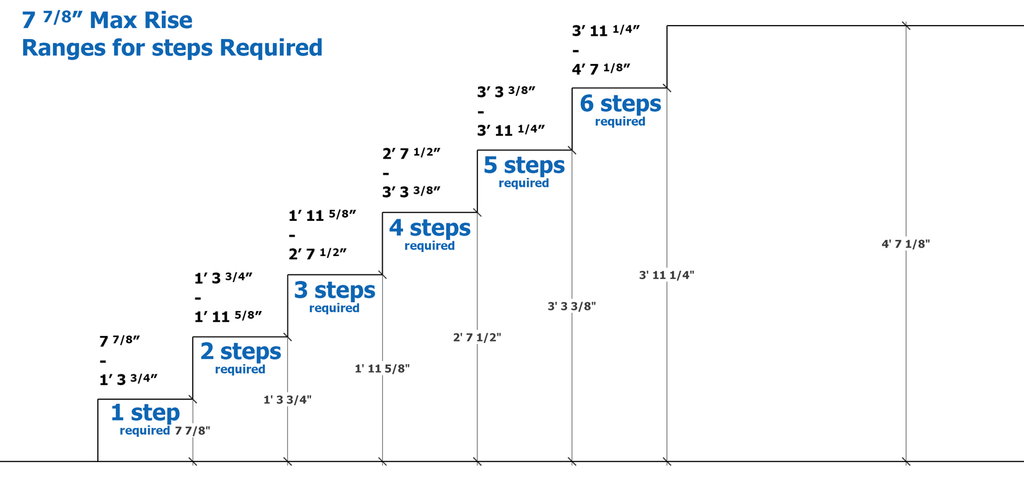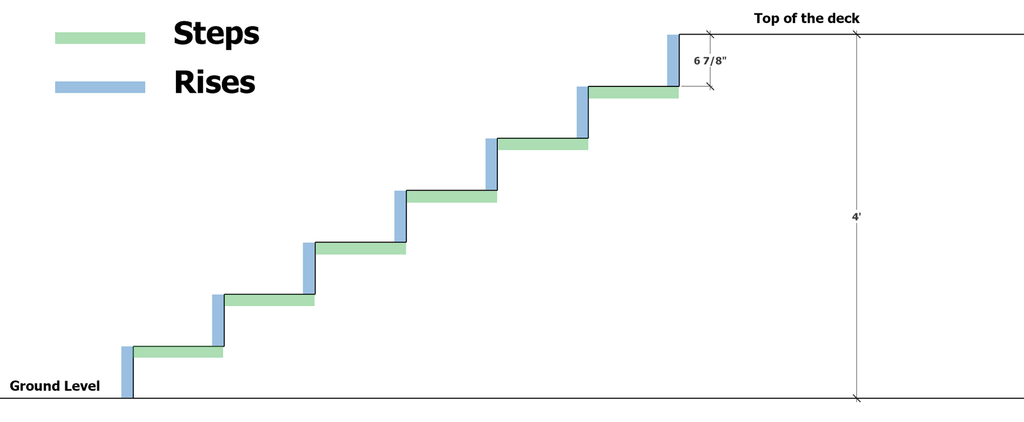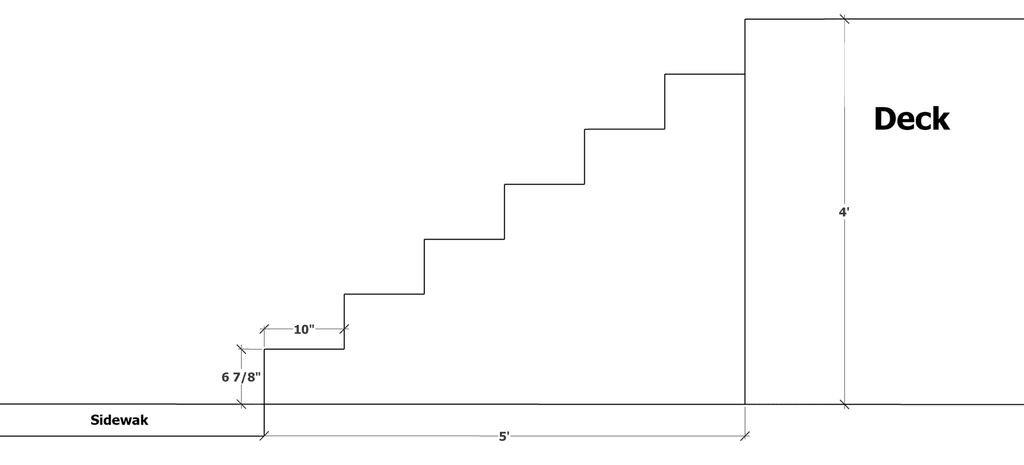# How many steps for my deck?

The number of steps affects your deck's price, how far your stairs reach into the yard and has some building code constraints. The more steps you have, the more deck boards and stair riser pieces you will need for your project. Depending on how wide, this can add hundreds of dollars for a single step. You will also want to follow the acceptable stair riser range of your local building codes.

Here is a quick reference diagram for some deck height ranges grouped by the number of steps. We are using Edmonton's maximum riser height.## Easy quick estimate

Deck Height ÷ 7.875

### Least amount of steps

In Edmonton, the maximum allowable stair rise is 7 7/8" or 7.875 in decimal form. To find the least number of steps for your deck, measure your deck height in inches at the stair location. Then divide by seven point eight seven five. You will get a whole or decimal number. Round down if it is a decimal and round up if it is a whole number.

To illustrate, if our deck height is 4 feet.

Convert this to inches (4 x 12" = 48")

Then divide by your largest allowable riser height

48 ÷ 7.875 = 6.10

Seven rises (roundup) with six steps (round down).

• We don't include the seventh rise because this is the final stepping height onto the deck. The deck is the step.
• One side of your stairs is often taller than the other. Always use the most elevated point for a stair calculation.## Maximum number of steps

A similar calculation, but instead, we round down for the riser total and minus one for the number of steps. Use your minimum riser height; if you roundup, this would push the riser under the minimum limit.

In Edmonton, the minimum is five inches.

48" ÷ 5" = 9.6

Nine rises (round down) with eight steps (minus one).
A four-foot high deck can have a range between 6 and 8 steps.

For a price comparison between our step range. If we had six-foot-wide stairs, this would be an additional \$64 in pressure treated decking material cost, not including framing or labour

or

Twelve-foot wide stairs using Deckorators composite.

\$482 more decking material.

### What is our riser height?

Consistent step height is essential for passing a deck inspection. The most common error made when building deck stairs is an inconsistent top and bottom step. Usually, this is because the builder forgot to account for the tread thickness. The first step to avoid this is an accurate, constant riser height.

Using the four-foot deck example, let's find the exact riser height.

To do this, divide the deck height by the number of stair rises and convert to inches.

48" ÷ 7 rises (1 more than our step quantity, we had five steps in our example) = 6.86"

Convert the .86 into a fraction by multiplying by the number of sixteenths in one inch.

.86 x 16 = 13.71 roundup = 14

The consistent riser height is 6 14/16"

or

6 7/8" (divide the fraction in half)

### Varying heights from the ground on a wide set of stairs.

Sometimes your stairs will land on an uneven surface. One side may be higher than the other. Use the tallest side of the stairs for your riser height and install the stair stringers from that side first. As you install more stringers and the ground clearance gets smaller, remove material from the bottom step. This is for safety and aesthetics. If a random step height causes a fall, you want it at the bottom of the stairs. And visually, decks have uniform shapes. When the shorter stair rises are at the bottom, the deck appears consistent. It's as though the deck was built first, and the landscape evolved around it.### Need the steps to end by a certain distance.

What if your stairs need to land on a patio or concrete sidewalk. You want it to be supported but not take up to much space. While at the same time, following your allowable step riser heights.

Here's how
Our tread width will decide how many steps we need. Let's say we have a concrete sidewalk that is five feet from the end of our four-foot high deck. And we want the last tread to end right at the edge of the sidewalk.We can have eight to six steps at that height, and in Edmonton, we have a tread width range of 9 1/4" - 14". Start by dividing the five-foot gap by one of our step counts to see if it falls in the tread width range. If it's too short or too long for our available step counts, consider changing how far your deck comes out.

Convert five-feet to inches (5 x 12" = 60")

Then divide by your largest amount of steps (8 in our example)

60 ÷ 8 (steps)= 7.5"

60 ÷ 7 (steps)= 8.57"

60 ÷ 6 (steps)= 10"

At a 5' distance six steps is our only option.Let's compare this tread to some deck board widths. If we are using pressure-treated lumber, our actual width of each board is 5 1/2".

Two deck boards 5.5" + 5.5" = 11"

If we want two solid boards, we will need a bigger gap than five-feet.

11" x 6 (steps)= 5' 6"Stair treads can also have a nosing (overhang). Suppose we want the bottom of the last step to butt into the sidewalk. So no dirt or grass gets in between the sidewalk and stairs. We need to minus the nosings overhang from the total gap.

1/2"(nosing) x 6(Steps) = 3"

5' 6" - 3" = 5' 3"• Edmonton allows up to 1" of nosing.
• Composite boards often have different widths than pressure-treated lumber and gaps between boards, which would also need to be added to the total tread width.

There are many other ways to find these stair counts and rise and runs.
Two of our favourite software to use for complex carpentry problems is Sketchup and FormIt. They both have a free online version.

Let us know if you have any decking questions, were here to help.